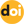The peer-reviewed scientific and technology journal. ISSN: 0204-3327

# GRS method to evaluate uncertainties in calculation parameters of an advanced fast reactorhttps://doi.org/10.26583/npe.2014.2.10

### UDC: 621.039.51.17

Evaluation of calculation errors due to uncertainties in neutron data and technological parameters (geometrical and material data) has become one of the most important problems of reactor physics. The well-known approach to solve this problem is based on the use of sensitivity coefficients of reactor calculation parameters of interest to the input data (neutron cross sections, geometrical and material data). The main advantage of this approach is that small computational times are necessary. On the other hand, there are certain drawbacks of the approach. Sensitivities are usually calculated by the first-order perturbation theory, so linear approximation is applied. Furthermore, different types of the perturbation theory should be used for different types of calculation parameters. The most commonly used type of the perturbation theory allows one to compute sensitivities of keff. Other modifications of the theory are more difficult to implement and use in 3D calculations. It is particularly difficult to calculate sensitivities of spatially distributed calculation parameters like power density.

Recently, because of the very high computational capabilities of modern computers, another approach has attracted the attention of reactor physicists. It is based on random sampling of input calculation data sets (neutron cross sections, geometrical and material data) and multiple recalculations of the reactor calculation parameters of interest. This results in sets of statistically distributed values for each calculation parameter. These sets can be then statistically processed to obtain mean values and variances of the calculation parameters.

This method is very easy to implement though multiple calculations imply that computational time could be considerable as compared with the sensitivity approach. On the other hand, in the GRS approach all types of calculation parameters are treated simultaneously and in the same manner: each run of a neutron transport code with randomly sampled input data yields random values of all the calculation parameters of interest, no matter how many such parameters are considered and which type each parameter belongs to. After a preset number of individual runs are performed, calculation uncertainties of the parameters are simultaneously estimated.

In this paper the GRS technique is applied to estimate the uncertainties of calculation parameters of an advanced sodium-cooled fast reactor, such as Keff, power density and stainless steel dose rate. These uncertainties are due to uncertainties of neutron cross sections and other input parameters of the reactor calculation model (geometrical and material data). The calculations were performed using the diffusion nodal code TRIGEX and Monte Carlo code MMK. Group constants were calculated by CONSYST on the basis of the 299-group ABBN library. In the case of keff, the results obtained with the GRS technique are compared with those obtained with the sensitivity approach.

1. Manturov G.N. Influence of Neutron Data Uncertainties on Accuracy of Prediction of Advanced Reactor Characteristics. - Proc. of Intern. Conf. Nuclear Data for Science and Technology, May 9-13 1994, Gatlinburg, Tennessee. Vol. 2, p.993-999 (1994), ORNL, ANS.
2. Zwermann W. et al. “Uncertainty Analyses with Nuclear Covariance Data in Reactor Core Calculations,” Int. Conf. on Nuclear Data for Science and Technology, Jeju Island, Korea, April 26-30, 2010.
3. Rochman D., Koning A.J., van der Marck S.C. Uncertainties for criticality-safety benchmarks and keff distributions, submitted to Annals of Nuclear Energy (2008).
4. Rochman D., Koning A.J., van der Marck S.C., van Veen D. Propagation of nuclear data uncertainty: Exact or with covariances, published by EDP Sciences (2010).
5. Manturov G.N., Nikolaev M.N., Tsiboulia A.M. “ABBN-93 Group Data Library. Part 1: Nuclear Data for the Calculations of Neutron and Photon Radiation Fields.” INDC (CCP)-409/L, IAEA, p. 65 (1997).
6. Seregin A.S., Kislitsina T.S., Tsiboulia A.M. Annotacija kompleksa program TRIGEX.04 [Description of the TRIGEX.04 Software Package]. Preprint IPPE-2846, Institute for Physics and Power Engineering, Obninsk (2000). (In Russian)
7. Blyskavka A.A., Manturov G.N., Nikolaev M.N., Tsibulya A.M. Programmnyj kompleks CONSYST/MMKKENO dlja rascheta jadernyh reaktorov metodom Monte-Karlo v mnogogruppovom priblizhenii s indikatrisami rassejanija v Pn-priblizhenii [CONSYST/ MMKKENO Software Package for Monte-Carlo calculation of nuclear reactors in the multi-group approximation with scattering indicators in Pn-approximation]. Preprint IPPE-2887, Institute for Physics and Power Engineering, Obninsk, 2001. (In Russian.)
8. Gantmaher F.R. Matrix Theory. Moscow. Nauka Publ. 1968. 576 p. (In Russian)
9. Poplavsky V.M., Tsiboulia A.M., Khomyakov Yu.S., Matveev V.I, Eliseev V.A., Tsikunov A.G., Vasiliev B.A., Belov S.B., Farakshin M.R. “Core Design and Fuel Cycle of Advanced Fast Reactor with Sodium Coolant.” Int. Conf. on Fast Reactors and Related Fuel Cycles: Challenges and Opportunities (FR09), Kyoto, Japan, December 7-11, 2009.